# VBA – Declare (Dim), Create, and Initialize Array Variable

This tutorial will demonstrate how to Declare (Dim), Create, and Initialize Array Variables in VBA

## What is a VBA Array Variable?

A VBA array variable can be thought of as a group of variables, stored under the same name and having the same data type. An array can store text, numbers, or objects.

You refer to an element in an array using its index number.

You can declare an array variable in the same way as you would declare any other variable by using the Dim, Static, Public or Private keyword.

## Static Arrays

There are 2 types of arrays – Static and Dynamic. A Static array is declared with it size specified when you initially declare the array. It is also called a Fixed Array.

``````Dim intA(4) as integer
``````

The above array is declared using the Dim statement at a Procedure or Module level, and the size of the array is 5 as we have not declared the LBound value of the array.

No, that’s not a typo! The Array size is 5, despite entering 4 into the array. This is because array indexes automatically start at zero.

### Array Indexes

Array indexes automatically begin at zero unless Option Base 1 is declared at the top of your code module.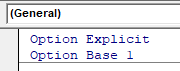If Option Base 1 is declared then arrays will automatically start at 1.

However, I find declaring variables in such a way to be problematic. Code reviewers may not be aware that arrays start at zero or of the Option Base 1 declaration

Instead, I prefer to explicitly declare the start and end positions of arrays:

``Dim intA(2 to 5) as integer``

Notice that when you do so, you can start the array at any number (not just 1 or 0).

## Dynamic Arrays

A Dynamic Array variable is an array whose size can be changed at runtime. You declare dynamic variables without a size.

``Dim intA() as integer``

You can use the ReDim statement to specify the size of the Array after the array has been created.

``ReDim intA(2)``

You can resize a dynamic array at any time. However, when using the ReDim Statement, all existing values are erased. To preserve existing array values, use ReDim Preserve instead.

``ReDim Preserve intA(2)``

You can declare a dynamic array at a Procedure, Module or Global level, but you can only use the ReDim statement within a Procedure.

## Variant Arrays

Variant arrays are Dynamic arrays that are easier to work with.

``Dim varNames()``

Notice that you don’t need to specify the data type (it is assumed to be variant) or the array size.

As we’ll see below, you can initialize variant arrays using the Array Function (no need to resize arrays first)!

## Declare Module & Public Arrays

As shown above, arrays can be declared within procedures, for use within that procedure:

``````Sub StaticArray()
'declare the array with an LBound value of 1 and an UBound value of 4
Dim IntA(1 to 4) as Integer
End Sub``````

But they can also be declared at the Module or Global level.

``````Option Explicit

'declare the array with an LBound value of 1 and an UBound value of 4
Dim IntA(1 to 4) as Integer

Sub StaticArray()
End Sub``````

In this example, the array variable can be called anywhere within this code module. Instead, you can declare a public array that can be used throughout your VBA Project (see next section).

Stop searching for VBA code online. Learn more about AutoMacro - A VBA Code Builder that allows beginners to code procedures from scratch with minimal coding knowledge and with many time-saving features for all users!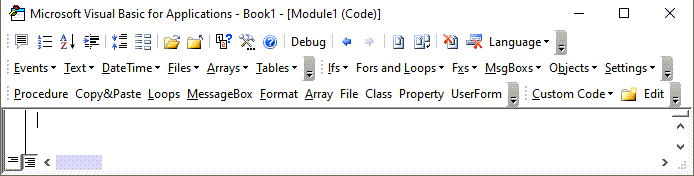### Declaring a Public Array

You declare a Public Static array as you would declare a Public Variable.

``Public strNames(3) as String``

This declaration would need to go at the top of your module, below Option Explicit.  It could then be used throughout your VBA project in any module or procedure.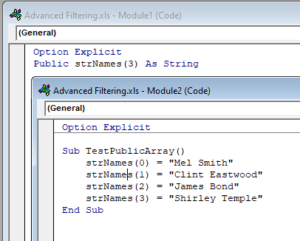If you declare the Array at the top of the module, but with the Dim keyword, then the use of that array is restricted to that individual module.  Trying to use the Array in a separate module would result in an error.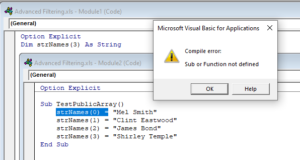## Initialize Arrays

You can assign values to a static array in the following way.

``````Sub StaticArray()
'declare the array with an LBound value of 1 and an UBound value of 4
Dim IntA(1 to 4) as Integer
'initialise the array
IntA(1) = 10
IntA(2) = 20
IntA(3) = 30
IntA(4) = 40
'show the result of position 2 of the array in the immediate window
Debug.Print IntA(2)
End Sub``````

If you run the above procedure,  the value of 20 would be displayed in the immediate window.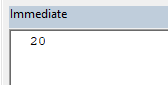You can also assign the values to a Dynamic Array in the same fashion

``````Sub DynamicArray()
'declare a dynamic array but omitting the bound values
Dim IntA() as Integer
'initialise the array
ReDim IntA(1 to 4)
IntA(1) = 10
IntA(2) = 20
IntA(3) = 30
IntA(4) = 40
'show the result of position 2 of the array in the immediate window
Debug.Print
IntA(2)
End Sub``````

### Array Function

However, with the variant array only, you can use the Array Function which may be easier than using the standard method.

``````'populate the array
intA() = Array(10, 20, 30, 40)``````

VBA Programming | Code Generator does work for you!

### Populate Array with Loop

You can also populate arrays by looping through a range of cells in Excel

``````Sub TestDynamicArrayFromExcel()
'declare the array
Dim strNames() As String
'declare an integer to count the rows in a range
Dim n As Integer
'declare an integer for the loop
Dim i As Integer
'count the rows in a the range
n = Range("A1", Range("A1").End(xlDown)).Rows.Count
'redim the array to the amount of rows in the range.
ReDim strNames(n)
For i = 0 To n
strNames(i) = Range("A1").Offset(i + 1, 0)
Next i
'show the values in the array
MsgBox Join(strNames())
End Sub``````

## Re-initialize Arrays

You can re-initialize your array at any stage of your code, but you will then lose the original value contained in that position in your array.

``````Sub StaticArray()
'declare the array with an LBound value of 1 and an UBound value of 4
Dim IntA(1 to 4) as Integer
'initialise the array
IntA(1) = 10
IntA(2) = 20
IntA(3) = 30
IntA(4) = 40
'show the result of position 2 of the array in the immediate window
Debug.Print IntA(2)
'initialize the array again
intA(2)= 200
Debug.Print IntA(2)
End Sub``````

In the example above, the Static array will keep all the values, except the value in position 2 – that value will change to 200.

### Using ReDim

If you are using a Dynamic Array, the ReDim statement is used to set the size of your Array.  You can use the ReDim statement subsequently in your code to change the size of the Array as many times as you need to.   The line of code below will re-initialize the intA Array to have a size of 2  (Remember – an Array index begins at 0!)

``ReDim intA(1) as Integer``

So the code including the ReDim statement would look like the example below.

``````Sub TestDynamicArray()
'declare the array
Dim intA() As Integer
ReDim intA(2)
'populate the array with numbers
intA(0) = 2
intA(1) = 5
intA(2) = 9
'show the number in position 1
Debug.Print intA(1)
'redim the array to change the size
ReDim intA(3)
intA(0) = 6
intA(1) = 8
'show the number in position 1 this time
Debug.Print intA(1)
End Sub``````

If  you run the above procedure,  the value of 5 would be displayed in the immediate window, and then a value of 8 would be displayed once we have resized the array using ReDim and repopulated it.  However, as we have not populated IntA(2), and we did not use Re-Dim Preserve, the value in that position in the array will be removed and both position 3 and 4 in the array will be zero.

### Using ReDim Preserve

If we use ReDim Preserve, it will keep the original values contained in the array.

``````Sub TestDynamicArray()
'declare the array
Dim intA() As Integer
ReDim intA(2)
'populate the array with numbers
intA(0) = 2
intA(1) = 5
intA(2) = 9
'show the number in position 2
Debug.Print intA(2)
'redim the array
ReDim Preserve intA(3)
intA(0) = 6
intA(1) = 8
'show the number in position 2 again
Debug.Print intA(2)
End Sub
``````

In both message boxes displayed above, the number 9 will appear as the ReDim Preserve statement kept that value in that position.